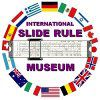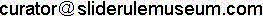HOME

### Gauge Marks Descriptions and values as located on the scalesKEEP ISRM FREE!
 Note: Thanks to Steve Seale Ph.D. who compiled this list of the most commonly found gauge marks. Nomenclature used here is consistent with that used in, "Pocket Book of the Gauge Marks", Panagiotis Venetsianos, The Oughtred Society, Roseville CA,, 2nd edition, 2011, 147 pp. Gauge marks in the table below are arranged in increasing order of their value

 Gauge Mark Value Definition Comments ρ or M 1.019 X 103 mils to Radians conversion Cu 1.124 X 10-1 finding weight of Cu solid s 1.273 4/π Flying Fish rules; finding area of circle C 1.128 sqrt(4/π) used to calculate circle areas and cylinder volumes m 1.28 used in US Gallon and meter conversions NP or dB 1.151 Ln(10)/20 Neper/decibel conversions '' or // or : 1182 X 104 (60*(180/π))² used to find sines or tangents of small angles N 1.340 used in converting British HP to/from kW DNM 1.359 Power conversion between Imperial and Metric units y 1.4 used in yard conversions Cu .1482 sqrt((.01722 X 4)/π) finding resistance of Copper wire Cu or Cu1 .1493 sqrt((.0175 X 4)/π) finding resistance of Copper wire Cu1 .1495 sqrt((0.01755 X 4)/π) finding resistance of Copper wire ρg 1.571 π/2 F or f .1592 1/(2π) for solving electrical circuit problems cm 16 Used in cm/inch conversions ' 1.667 X 10-2 1 minute = 1°/60 converts minutes to radians 1.732 √3 Used in solving 3-φ problems Q or ρ or ρ0 or 1° 1745 X 10-5 π/180 used to find sines or tangents of small angles ρCu 1.750 X 10-2 finding resistance of Copper wire d 1.772 sqrt(π) Cu 1.785 X 10-2 finding resistance of a Copper wire v or ρ 1.910 6/π finding volume of sphere Al1 or Al .1922 sqrt((.029 X 4)/π) finding resistance of Aluminum wire 2g 19.62 Twice the gravitational constant ' or / or · 1970 X 10² 60*(180/π)² used to find sines or tangents of small angles R1 1.986 Molecular Gas Constant in CGS units ρ'' or '' or S or R'' or Q'' 2063 X 10² (180*60*60)/π used to find sines and tangents of small angles c 2.154 ∛10 used for finding cube roots when rule has no K scale V0 or VM or MV 22.42 litrs/mol ideal gas ideal gas calculations 1n or L or U or 1/M 2.303 1/Log10e Used for converting between natural and common logs kg 245 Used in lb/kg conversions f 2.533 X 10-2 1/(4 X π)² Resonant frequency calculations F or 1F 26.8 Faraday Constant γAl 2.700 finding weight of Aluminum wire e 2.718 Euler number; a constant Base of Natural Logarithms '' 2.778 X 10-4 1 second = 1°/3600 converts seconds to radians ℧ or V or 1/R 28.70 finding the voltage drop in Copper wires ρAl 2.900 X 10-2 finding resistance of Aluminum wire ' or 1/ρ' 2.908 X 10-4 π/(180 X 60) finding sines of small amgles expressed in minutes W 3.027 x 10-3 finding wt. of Cu wire given dia. and length V 30.49 23.950 X 4/π finding voltage drop in Cu conductors π 3.142 Ratio of circumference to diameter of a circle □ 3.162 √10 M .3183 reciprocal of π gE or g 32.19 Gravitational Constant W 3.318 X 105 sqrt((4 X 44 X 106)/(π X 3 X 555)) finding weight of Copper wire ρ' or ' or m or R' or Q' 3438 (180*60)/π used to find sines and tangents of small angles Al 35.0 finding the resistance of Aluminum wire C1 or C' 3.568 sqrt(40/π) used to calculate circle areas K .3592 273/760 gas volume calculations 1/e .3679 Reciprocal of e Cu .3782 sqrt(4/(π X 8.9)) for weight calculations of round Copper wire Cu2 .3784 sqrt(4/(π X 8.89)) for weight calculations of round Copper wire 39.4 .3937 1/(2.54) used in in/cm conversions J 4.186 No. of Joules in a calorie, or Watts in a calorie/sec V 4.189 (4 X π)/3 finding volume of a sphere; usually on K scale M 4.27 X 10² 427 Kg-M = 1 Kcal M .4343 Log10e √ 4.429 sqrt(2g) or sqrt(2 X 9.81) used in hydraulic problems N .4492 1.2505 x (273/760) ideal gas calculations c2 4.642 ∛100 used for finding cube roots when rule has no K scale kW 465 Coupled Mark for kilowatt conversions '' or 1/ρ'' 4.848 X 10-6 π/(180 X 60 X 60) finding sines of small angles expressed in seconds XM .5235 for computing reduction to the meridian V .5236 π/6 finding volume of a sphere 2F 53.58 2 X Faraday Constant lbs 54 Used in lb/avoirdupois conversions R 552.8 sqrt(40/π X 24000) finding resistance of Copper wire Cu 57.1 finding the resistance of Copper wire Qo or ρ0 or ρ or R or r or U or Ro 5.730 X 101 180/π used to find sines and tangents of small angles; to convert Degrees to Radians 60° .5774 tan of 30° or 1/(sqrt(3)) used for 1/(sqrt(3)) or 3φ current calclations R2 62.36 Molecular Gas Constant in 63 Used in in/cm conversions PS 632 Coupled mark for metric horsepower conversions Q,, or ρ,, or ρ or ρ, or ρg 6366 X 10² (200*100*100)/π used to find sines and tangents of small angles Al2 .6880 sqrt(4/(π X 2.69)) weight calculations of round Aluminum wire N .7136 sqrt((.4 X 4)/π) finding resistance in Nickelin wire PS or MOT .7358 1 metric horsepower = .73575 kilowatt converting horsepower to kilowatts M .738 Probably finding resistance in Manganin wire E or e 775 conveting Watts to HP HP or W .7456 1 British horsepower = .7456 kilowatt converting horsepower to kilowatts A or S .7854 π/4 calculates area of circle from diameter K .798 Probably finding resistance in Constantan wire 3F 80.4 3 X Faraday Constant R3 8.205 X 10-2 Molecular Gas Constant in ISO units dB 8.686 20/Ln(10) Neper/decibel conversion γCu 8.900 finding the weight of Copper wire Y 0.9144 # meters/yard for meters/yards conversion gF 9.81 Gravitational Constant
 Copyright © 2003- International Slide Rule Museum